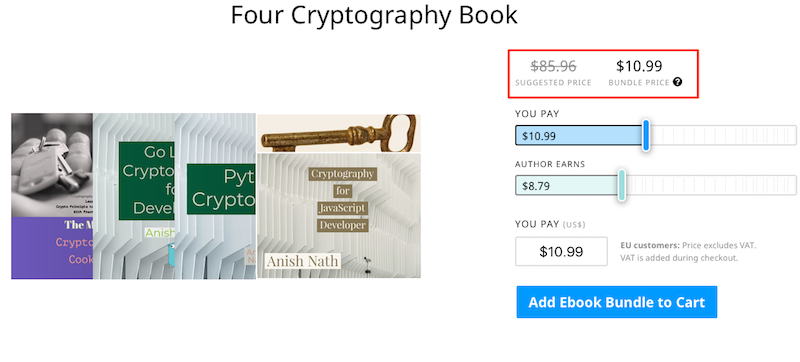# Web Crypto API Digital Signature Using PBKDF2 and HMAC

by Anish

Posted on Tuesday september 25, 2018

This sample chapter extracted from the book, Cryptography for JavaScript Developers.

In this example we are going to produce the digital signature of the message by using pbkdf2 key derivation function, and then sign the message from the derived key using HMAC, then we are going to verify the message

## PBKDF2,HMAC Digital Signature Demo

This is the web cryptography api example of producing digital signature of the message

Iteration:
Input Message:
Singed Message:
PBKDF2 Derived Key (Hex):
Randow Salt (Hex) :

## pbkdf2,hmac Javascript example of using webcrypto api

The first step is to derive the key from the user supplied password,

``````window.crypto.subtle.importKey(
'raw',
passphraseKey,
{name: 'PBKDF2'},
false,
['deriveBits', 'deriveKey']
).then(function(key) {

return window.crypto.subtle.deriveKey(
{ "name": 'PBKDF2',
"salt": saltBuffer,
"iterations": iterations,
"hash": 'SHA-256'
},
key,
{ "name": 'HMAC', hash: {name: "SHA-256"} },
true,
[ "sign","verify"]
)
}).then(function (webKey) {
publicKey=webKey
``````

Once the key is derived, now we are signing the message, using this derived key

``````window.crypto.subtle.sign(
{
name: "HMAC"
},
webKey,
asciiToUint8Array(plainText) //ArrayBuffer of data we want to sign
)
.then(function(signature){
document.getElementById("signature").value= bytesToHexString(signature)
})
.catch(function(err){
console.error(err);
});

return crypto.subtle.exportKey("raw", webKey);

}).then(function (buffer) {

document.getElementById("key").value= bytesToHexString(buffer);
document.getElementById("salt").value= bytesToHexString(saltBuffer);
});``````

Signature Verification To verify the signature of the message, pass the pbdkf2 crypto key,signature and the plain text message

``````window.crypto.subtle.verify({
name: "HMAC",
},
publicKey,
hexStringToUint8Array(signature),
asciiToUint8Array(plainText)
)
.then(function(decrypted) {
})
.catch(function(err) {
console.error(err);
});``````

Thanku for reading !!! Give a Share for Support

Asking for donation sound bad to me, so i'm raising fund from The Modern Cryptography CookBook for Just \$9. Leanpub books Discount coupon first 100 reader. No hurry read the sample chapters here then decide.

Alternatively to support you can buy My all four Cryptography book Just \$10.99• The Modern Cryptography Book.
• Go lang Cryptography for developers
• Python Cryptography
• Cryptography for JavaScript Developer

Here